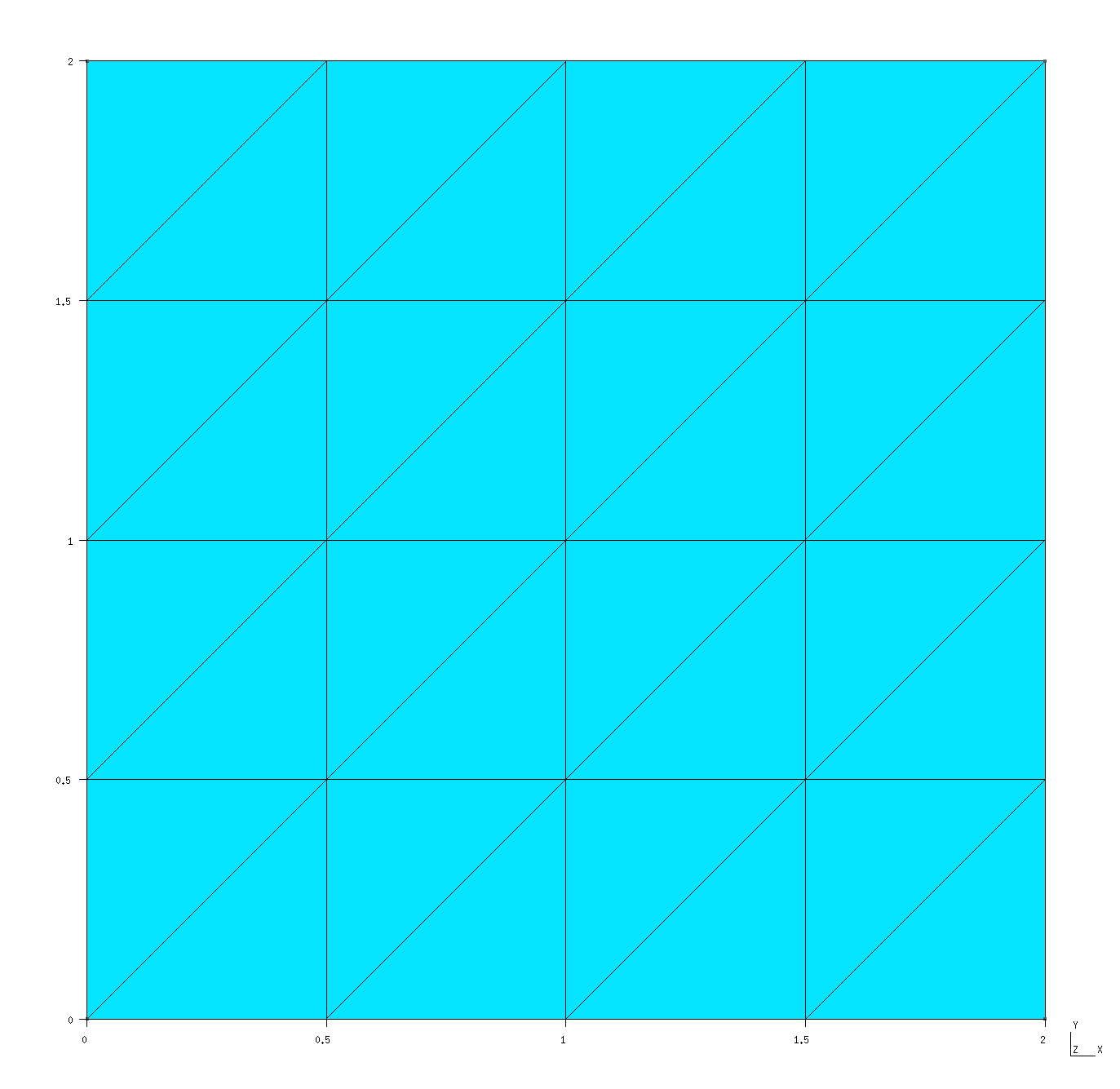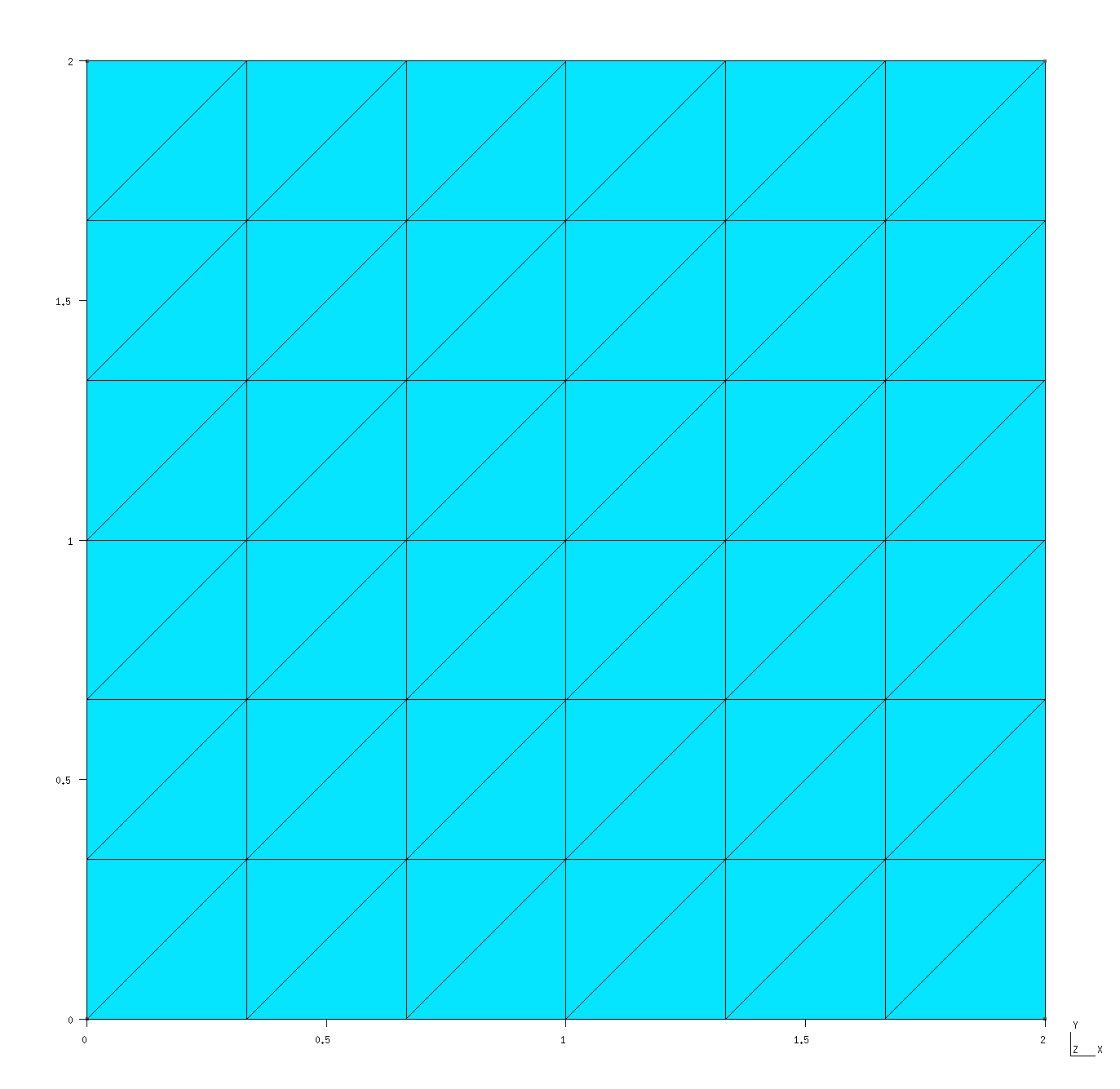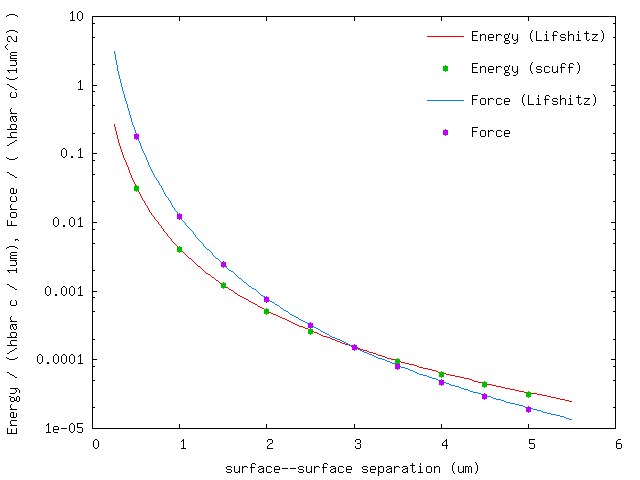Casimir forces between infinite-area silicon slabs (2D periodicity)

In this example, we exploit scuff-em's support for 2D periodic geometries to compute the equilibrium Casimir force per unit area between silicon slabs of infinite surface area. The files for this example may be found in the share/scuff-em/examples/SiliconSlabs subdirectory of your scuff-em installation.

gmsh geometry file for unit-cell geometry

The gmsh geometry file Square_N.geo describes the portion of the surface of a single slab that lies within the unit cell, i.e. the cell that is infinitely periodically replicated to yield the full geometry. In this case, the slab is infinitely thick (it is a half-space), so its surface consists of just a single two-dimensional sheet extending throughout the entire unit cell. I call this file Square_N.geo to remind myself that it contains a parameter N that describes the meshing fineness; more specifically, N defines the number of segments per unit length.

To produce a discretized surface-mesh representation of this geometry, we run it through gmsh:

% gmsh -2 Square_N.geo


This produces the file Square_N.msh, which I rename to Square_L2.40.msh because the side length of the square is $L=2\,\mu\text{m}$ and because this particular mesh has 40 interior edges (this number defines the number of surface-current basis functions and thus the size of the BEM matrix in a scuff-em calculation). Editing the .geo file to change the N parameter to 3 (from its default value of 2) and re-running gmsh -2 produces a finer mesh file, which I rename to Square_L2_96.msh. These meshes may be visualized in gmsh:

% gmsh Square_L2_40.msh
% gmsh Square_L2_96.msh


Square_L2_40.mshSquare_L2_96.mshNote the following:

• For 2D periodic geometries in scuff-em, the lattice vectors must lie in the $xy$ plane.

• For surfaces that straddle the unit-cell boundaries (as is the case here), each triangle edge that lies on any edge of the unit cell must have an identical image edge on the opposite side of the unit cell. An easy way to achieve this is to use extrusions in gmsh, as in the .geo file above.

• In this case the unit cell dimensions are $L_x\times L_y$ where $L_x=L_y=2\, \mu\text{m}$. (More generally, $L_x$ and $L_y$ may be any arbitrary nonzero values, and they need not equal each other.)

scuff-em geometry file

The scuff-em geometry file describing two silicon half-spaces (infinitely-thick slabs) bounded by the coarser of the two unit-cell meshes described above is SiliconSlabs_L2_40.scuffgeo:

# SCUFF-EM geometry file for two silicon slabs,
# each of infinite cross-sectional area and infinite
# thickness, separated in the z direction by 1 microns

LATTICE
VECTOR 2.0 0.0
VECTOR 0.0 2.0
ENDLATTICE

MATERIAL SILICON
epsf = 1.035;      # \epsilon_infinity
eps0 = 11.87;      # \epsilon_0
wp = 6.6e15;       # \plasmon frequency
Eps(w) = epsf + (eps0-epsf)/(1-(w/wp)^2);
ENDMATERIAL

REGION UpperSlab MATERIAL Silicon
REGION Gap       MATERIAL Vacuum
REGION LowerSlab MATERIAL Silicon

SURFACE UpperSurface
MESHFILE Square_L2_40.msh
REGIONS UpperSlab Gap
DISPLACED 0 0 0.5
ENDOBJECT

SURFACE LowerSurface
MESHFILE Square_L2_40.msh
REGIONS LowerSlab Gap
ENDOBJECT


Note the following points:

scuff-em transformation file

The file describing a list of geometric transformations is Slabs.trans:

TRANS 0.5 SURFACE UpperSurface DISP 0 0 0.0
TRANS 1.0 SURFACE UpperSurface DISP 0 0 0.5
TRANS 1.5 SURFACE UpperSurface DISP 0 0 1.0
TRANS 2.0 SURFACE UpperSurface DISP 0 0 1.5
TRANS 2.5 SURFACE UpperSurface DISP 0 0 2.0
TRANS 3.0 SURFACE UpperSurface DISP 0 0 2.5
TRANS 3.5 SURFACE UpperSurface DISP 0 0 3.0
TRANS 4.0 SURFACE UpperSurface DISP 0 0 3.5
TRANS 4.5 SURFACE UpperSurface DISP 0 0 4.0
TRANS 5.0 SURFACE UpperSurface DISP 0 0 4.5


It's convenient to visualize the effect of these transformations on our geometry before we run the calculation:

% scuff-analyze --geometry SiliconSlabs_L2_40.scuffgeo --TransFile Slabs.trans


This produces the file SiliconSlabs_L2_40.transformed.pp, which can be visualized by opening it in GMSH:Launching the run

Here's a bash script that will run the full Casimir calculation on both the coarser and finer meshes:

# /bin/bash

for N in 40 192
do
ARGS=""
ARGS="${ARGS} --geometry SiliconSlabs_L2_${N}.scuffgeo"
ARGS="${ARGS} --TransFile Beams.trans" ARGS="${ARGS} --BZSymmetry BZSymmetry"
ARGS="${ARGS} --energy" ARGS="${ARGS} --zForce"

scuff-cas3D \${ARGS}
done


This produces the files SiliconSlabs_L2_40.out and SiliconSlabs_L2_96.out. The former file looks like this:

# scuff-cas3D run on superhr1 at 06/15/15::23:50:32
# data file columns:
#1: transform tag
#2: energy
#3: energy error due to numerical Xi integration
#4: z-force
#5: z-force error due to numerical Xi integration
0.5 -1.254502e-01 5.463886e-05 -7.264755e-01 3.268714e-04
1.0 -1.631064e-02 5.770642e-06 -4.865678e-02 2.055737e-05
...


Now plot the energy and force per unit area vs. separation distance:

% gnuplot
gnuplot> set xlabel 'surface--surface separation (um)'
gnuplot> set ylabel 'Energy / (\hbar c / 1um), Force / ( \hbar c/(1um^2) )'
gnuplot> set logscale y
gnuplot> FILE='SiliconSlabs_L2_N40.out'
gnuplot> plot FILE u 1:2 t 'Energy (SCUFF)', '' u 1:4 t 'Force (SCUFF)'In this plot, the solid lines are the results of the Lifshitz formula for the Casimir energy and force between silicon slabs.